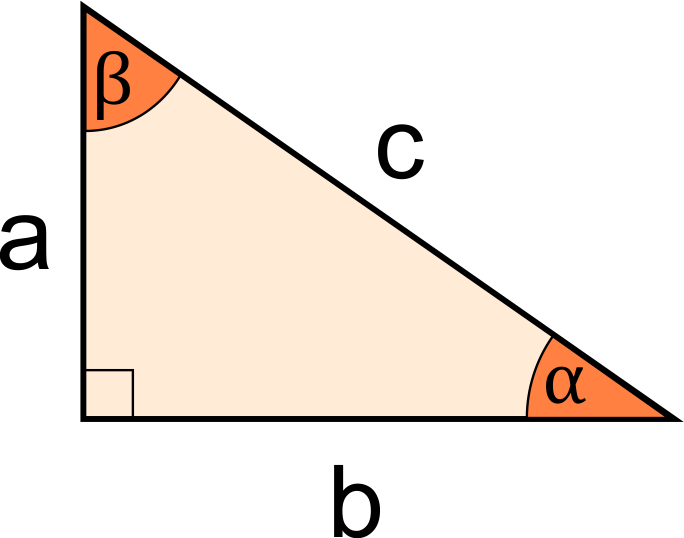# ABC Triangle Calculator

Reviewed by Rahul Dhari
Last updated: Jun 28, 2022

Using the ABC triangle calculator, you can calculate the right triangle sides, or calculate the right triangle angles. From the known values of sides / angles / area, you will be able to find all missing measurements in the right triangle!

## What is a right triangle?

A right triangle is any triangle that satisfies the Pythagorean theorem. As per the Pythagorean theorem, the square of the largest side must be equal to the sum of squares of the other two sides in a right triangle. Any triangle that satisfies this condition will be a right-angled triangle.

For example, consider a triangle with side lengths 3, 4 and 5. Here, the square of the largest side (5) is 25. The sum of squares of the other 2 sides is 9 + 16, which also gives us 25. Therefore, a triangle with side lengths 3, 4 and 5 units will be a right-angled triangle, and these numbers (3, 4, 5) are said to form a Pythagorean triplet.

## How do I use the ABC triangle calculator?

To use the ABC triangle calculator, you need to know one of the following, for a right triangle:

• Two sides of the right triangle;
• One acute angle and one side of the right triangle; or
• Area and one side of the right triangle.

Depending on your available measurements, do the following:

1. Choose a preferred combination of input measurements.
2. Key in the values.
3. The ABC triangle calculator will automatically calculate all the other unknown measurements in the triangle!

## Other right triangle calculators

If you found this tool useful in calculating the right triangle angles and sides, you might also want to take a look at some of our other right triangle calculators listed below:

## FAQ

### Does 4-5-6 make a right triangle?

No, a triangle with side lengths 4, 5 and 6 units will not form a right triangle, because these numbers don't satisfy the Pythagorean theorem. We can verify it as follows:

1. The square of the largest side (6) is 36.
2. The square of 4 is 16.
3. The square of 5 is 25.
4. The sum of 16 and 25 is 41, which is not equal to 36. So these lengths will not form a right-angled triangle.

### Is 1-2-3 a Pythagorean triplet?

No, the numbers 1, 2, and 3 do not form a Pythagorean triplet because they fail the condition for the Pythagorean theorem. The sum of squares of 1 and 2 (1 + 4) is 5, and it is not equal to the square of 3 (9).

Given
two sidesa
in
b
in
c
in
Angle α
deg
Angle β
deg
People also viewed…

### Exterior angles of a triangle

Use the exterior angles of a triangle calculator to determine the exterior angles of a triangle.

### Plastic Footprint

Find out how much plastic you use throughout a year with this plastic footprint calculator. Rethink your habits, reduce your plastic waste, and make your life a little greener.

### Podcasts

Do you feel like you could be doing something more productive or educational while on a bus? Or while cleaning the house? Well, why don't you dive into the rich world of podcasts! With this podcast calculator, we'll work out just how many great interviews or fascinating stories you can go through by reclaiming your 'dead time'!